### Potentiometers explained

A potentiometer is a device that allows you to produce any potential / voltage up to the maximum of the supply. It works on the same idea as a potential divider except that the resistors used in this device can be changed. If you have not covered the use of a potential divider then don’t worry, it is not complicated, there is an explanation with a video here.

To explain how a potentiometer works suppose we have a long wire with a significant resistance or length of a graphite compound (like a pencil lead) and connect it into a circuit like this: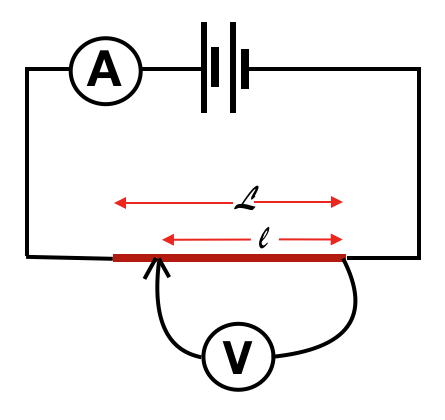Linear potentiometer

The potential difference across the resistance material (in red) will be the whole of that of the supply. But if we connect the voltmeter, as shown below, across half of the length, the value we measure on that will be half the total. (We are assuming the wire/graphite stick is uniform)

If we make the contact a quarter of the way along or three quarters then the potential difference will be one quarter or three quarters. We can produce whatever PD we like from zero up to the maximum of the supply. To give this as an equation:

The voltage provided equals:

the supply voltage x the length between the terminals divided by the total length.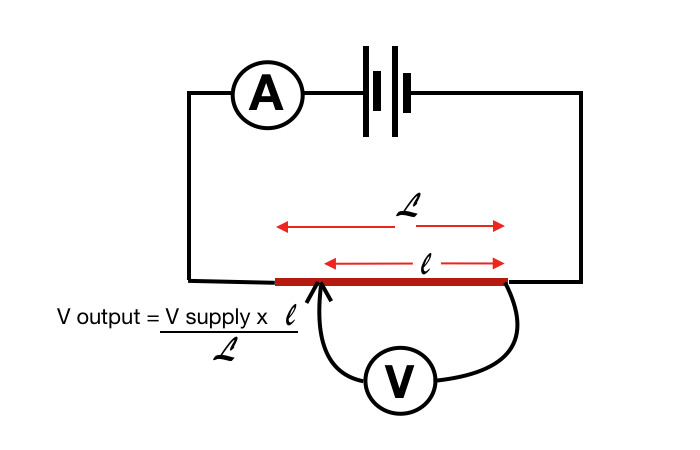Linear potentiometer equation

Commercial potentiometers like this are available but are largely used in science labs. The vast majority have to be compact so they fit into small electronic devices.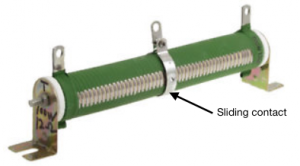Tubular potentiometer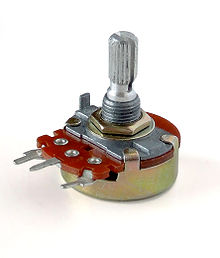Small compact potentiometer

Even though they are much smaller they work in the same way. The wire coil or graphite strip is shaped into a large arc as you see below. The sliding contact can then be twisted around the arc so changing the length of the material in the circuit.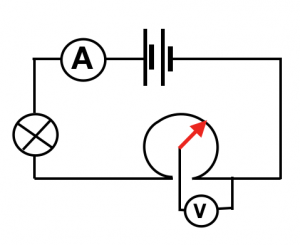Pictorial image of a small rotational potentiometer

The circuit symbol for a potentiometer is a box with an arrow pointing to it: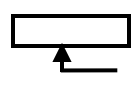Potentiometer symbol

and so the circuit diagram would be: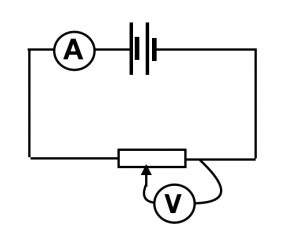Formal potentiometer circuit diagram

The video below should explain this more simply.

Related pages on potential difference / voltage, all with video lessons are here: